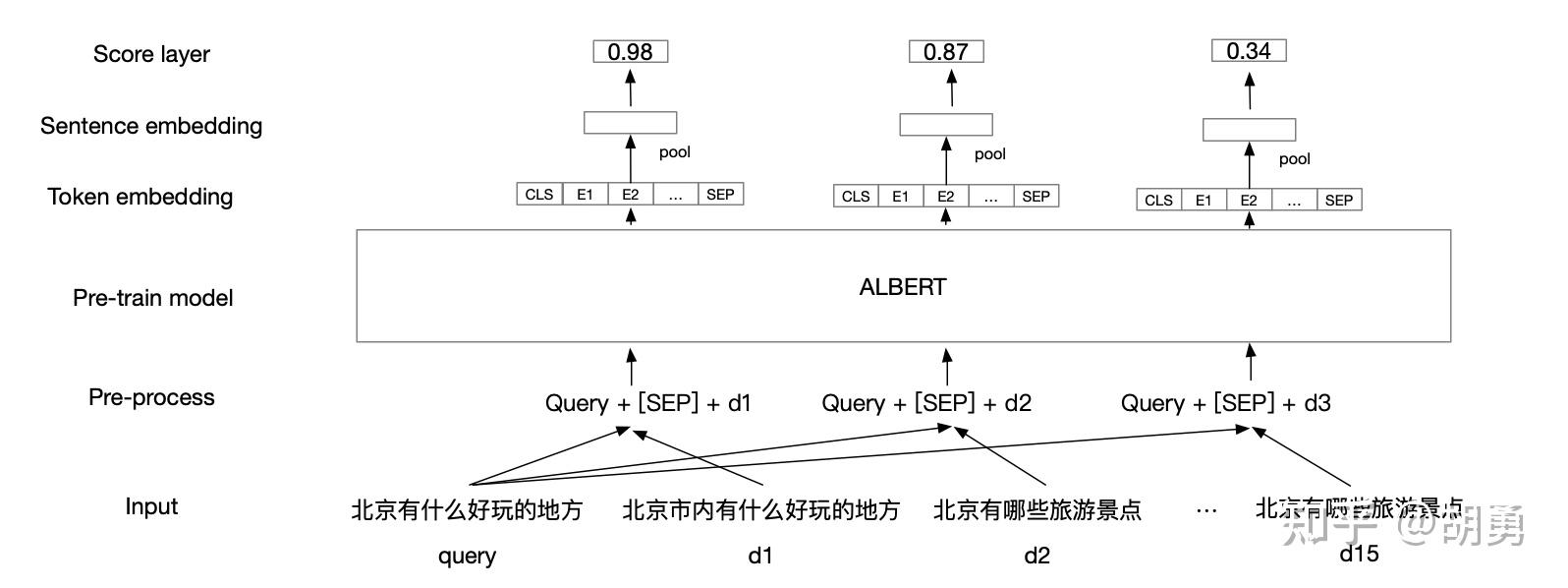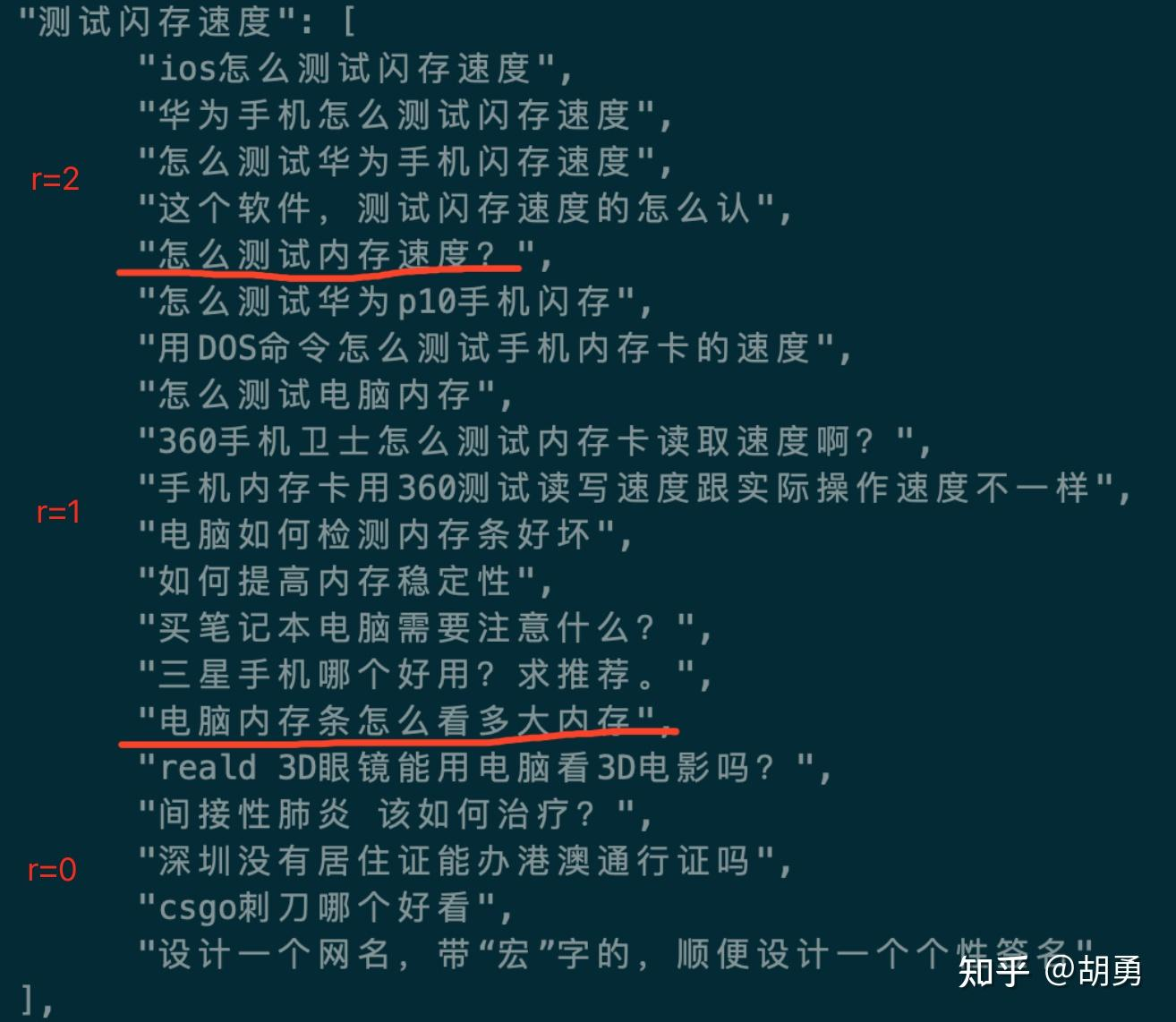# 任务描述

• (1) 如果top1的评分”很高”，则直接将答案返回给用户
• (2) 如果top1的评分”较高”，则进行问题的推荐
• (3) 如果top1的评分”较低”，则提示无法回答。

# 评价指标

Gain(G)表示$q$与检索结果$d$之间的相关性，计为$r$。在问答场景里面我们定义三种级别：

q与d的相关度描述 r

Cumulative Gain(CG) 表示对$top@K$个相关性的加和:$CG@K=\sum_{i=1}^{K}r(i)$

Discounted Cumulative Gain(DCG) 继续引入位置的特征，排在前面的元素增益应该更高，排在后面的元素增益应该相对较低: $DCG@K=\sum_{i=1}^{K}\frac{2^{r(i)}-1}{\log_{2}(i+1)}$

Normalized Discounted Cumulative Gain (NDCG): 若对于$q$最佳的$top@K$ 结果为$\{ {d_{1}^{‘},d_{2}^{‘},..,d_{k}^{‘}} \}$, 记q与最佳序列的DCG为IDCG, 即:$IDCG=DCG_{max}@K$ , 那么$NDCG=\frac{DCG@K}{IDCG@K}$。

# 模型结构$L=\sum_{r(i)>r(j)}|\Delta NDCG|\log_{2}(1+e^{-\sigma*(s_{i}-s_{j})})$

# 数据集构建

$$\{q,d_{1-1},d_{1-2},..,d_{1-5},d_{2-1},d_{2-2},..,d_{2-10},d_{3-1},d_{3-2},..,d_{3-5}\}$$